Skip to content
Related Articles
Convert Floats to Integers in a Pandas DataFrame
• Last Updated : 20 Aug, 2020

Let us see how to convert float to integer in a Pandas DataFrame. We will be using the `astype()` method to do this. It can also be done using the `apply()` method.

Method 1: Using `DataFrame.astype()` method

First of all we will create a DataFrame:

 `# importing the library``import` `pandas as pd`` ` `# creating a DataFrame``list` `=` `[[``'Anton Yelchin'``, ``36``, ``75.2``, ``54280.20``], ``        ``[``'Yul Brynner'``, ``38``, ``74.32``, ``34280.30``], ``        ``[``'Lev Gorn'``, ``31``, ``70.56``, ``84280.50``],``        ``[``'Alexander Godunov'``, ``34``, ``80.30``, ``44280.80``], ``        ``[``'Oleg Taktarov'``, ``40``, ``100.03``, ``45280.30``],``        ``[``'Dmitriy Pevtsov'``, ``33``, ``72.99``, ``70280.25``], ``        ``[``'Alexander Petrov'``, ``42``, ``85.84``, ``25280.75``]]``df ``=` `pd.DataFrame(``list``, columns ``=``[``'Name'``, ``'Age'``, ``'Weight'``, ``'Salary'``])``display(df)`

Output :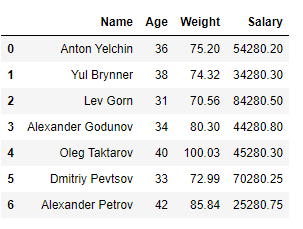Example 1 : Converting one column from float to int using `DataFrame.astype()`

 `# displaying the datatypes``display(df.dtypes)`` ` `# converting 'Weight' from float to int``df[``'Weight'``] ``=` `df[``'Weight'``].astype(``int``)`` ` `# displaying the datatypes``display(df.dtypes)`

Output :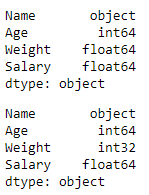Example 2: Converting more than one column from float to int using `DataFrame.astype()`

 `# displaying the datatypes``display(df.dtypes)`` ` `# converting 'Weight' and 'Salary' from float to int``df ``=` `df.astype({``"Weight"``:``'int'``, ``"Salary"``:``'int'``}) `` ` `# displaying the datatypes``display(df.dtypes)`

Output :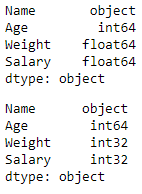Method 2: Using `DataFrame.apply()` method

First of all we will create a DataFrame.

 `# importing the module``import` `pandas as pd`` ` `# creating a DataFrame``list` `=` `[[``15``, ``2.5``, ``100.22``], [``20``, ``4.5``, ``50.21``], ``        ``[``25``, ``5.2``, ``80.55``], [``45``, ``5.8``, ``48.86``], ``        ``[``40``, ``6.3``, ``70.99``], [``41``, ``6.4``, ``90.25``], ``        ``[``51``, ``2.3``, ``111.90``]]``df ``=` `pd.DataFrame(``list``, columns ``=` `[``'Field_1'``, ``'Field_2'``, ``'Field_3'``],``                  ``index ``=` `[``'a'``, ``'b'``, ``'c'``, ``'d'``, ``'e'``, ``'f'``, ``'g'``])``display(df)`

Output :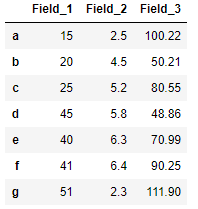Example 1: Converting a single column from float to int using `DataFrame.apply(np.int64)`

 `# importing the module``import` `numpy as np`` ` `# displaying the datatypes``display(df.dtypes)`` ` `# converting 'Field_2' from float to int``df[``'Field_2'``] ``=` `df[``'Field_2'``].``apply``(np.int64)`` ` `# displaying the datatypes``display(df.dtypes)`

Output :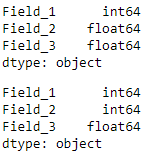Example 2: Converting multiple columns from float to int using `DataFrame.apply(np.int64)`

 `# displaying the datatypes``display(df.dtypes)`` ` `# converting 'Field_2' and 'Field_3' from float to int``df[``'Field_2'``] ``=` `df[``'Field_2'``].``apply``(np.int64)``df[``'Field_3'``] ``=` `df[``'Field_3'``].``apply``(np.int64)`` ` `# displaying the datatypes``display(df.dtypes)`

Output :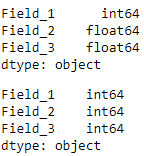Attention geek! Strengthen your foundations with the Python Programming Foundation Course and learn the basics.

To begin with, your interview preparations Enhance your Data Structures concepts with the Python DS Course. And to begin with your Machine Learning Journey, join the Machine Learning – Basic Level Course

My Personal Notes arrow_drop_up